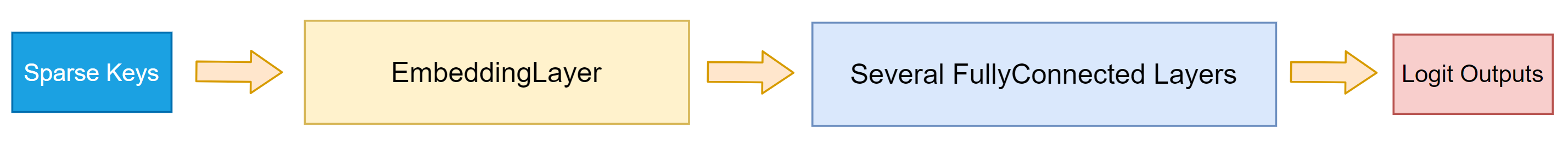# Get Started With SparseOperationKit

This document will walk you through simple demos to get you familiar with SparseOperationKit.

For experts or more examples, please refer to Examples section

Important

In this document and other examples in SOK, you are assumed to be familiar with TensorFlow and other related tools.

## Install SparseOperationKit

Please refer to the Installation section to install SparseOperationKit to your system.

## Import SparseOperationKit

import sparse_operation_kit as sok


## Define a model with TensorFlow

The structure of this demo model is depicted in Fig 1.Fig 1. The structure of demo model

import tensorflow as tf

class DemoModel(tf.keras.models.Model):
def __init__(self,
max_vocabulary_size_per_gpu,
slot_num,
nnz_per_slot,
embedding_vector_size,
num_of_dense_layers,
**kwargs):
super(DemoModel, self).__init__(**kwargs)

self.max_vocabulary_size_per_gpu = max_vocabulary_size_per_gpu
self.slot_num = slot_num            # the number of feature-fileds per sample
self.nnz_per_slot = nnz_per_slot    # the number of valid keys per feature-filed
self.embedding_vector_size = embedding_vector_size
self.num_of_dense_layers = num_of_dense_layers

# this embedding layer will concatenate each key's embedding vector
self.embedding_layer = sok.All2AllDenseEmbedding(
max_vocabulary_size_per_gpu=self.max_vocabulary_size_per_gpu,
embedding_vec_size=self.embedding_vector_size,
slot_num=self.slot_num,
nnz_per_slot=self.nnz_per_slot)

self.dense_layers = list()
for _ in range(self.num_of_dense_layers):
self.layer = tf.keras.layers.Dense(units=1024, activation="relu")
self.dense_layers.append(self.layer)

self.out_layer = tf.keras.layers.Dense(units=1, activation=None)

def call(self, inputs, training=True):
# its shape is [batchsize, slot_num, nnz_per_slot, embedding_vector_size]
emb_vector = self.embedding_layer(inputs, training=training)

# reshape this tensor, so that it can be processed by Dense layer
emb_vector = tf.reshape(emb_vector, shape=[-1, self.slot_num * self.nnz_per_slot * self.embedding_vector_size])

hidden = emb_vector
for layer in self.dense_layers:
hidden = layer(hidden)

logit = self.out_layer(hidden)
return logit


## Use SparseOperationKit with tf.distribute.Strategy

SparseOperationKit is compatible with tf.distribute.Strategy. More specificly, tf.distribute.MirroredStrategy and tf.distribute.MultiWorkerMirroredStrategy.

### with tf.distribute.MirroredStrategy

Documents for tf.distribute.MirroredStrategy. tf.distribute.MirroredStrategy is a tool to support data-parallel synchronized training in single machine, where there exists multiple GPUs.

Caution

The programming model for MirroredStrategy is single-process & multi-threads. But due to the GIL in CPython interpreter, it is hard to fully leverage all available CPU cores, which might impact the end-to-end training / inference performance. Therefore, MirroredStrategy is not recommended for multiple GPUs synchronized training.

create MirroredStrategy

strategy = tf.distribute.MirroredStrategy()


Tip

By default, MirroredStrategy will use all available GPUs in one machine. If you want to specify how many GPUs are used or which GPUs are used for synchronized training, please set CUDA_VISIBLE_DEVICES.

create model instance under MirroredStrategy.scope

global_batch_size = 65536
use_tf_opt = True

with strategy.scope():
sok.Init(global_batch_size=global_batch_size)

model = DemoModel(
max_vocabulary_size_per_gpu=1024,
slot_num=10,
nnz_per_slot=5,
embedding_vector_size=16,
num_of_dense_layers=7)

if not use_tf_opt:
else:



For a DNN model built with SOK, sok.Init must be used to conduct initilizations. Please see its API document.

define training step

loss_fn = tf.keras.losses.BinaryCrossentropy(from_logits=True, reduction=tf.keras.losses.Reducetion.NONE)
def _replica_loss(labels, logits):
loss = loss_fn(labels, logits)
return tf.nn.compute_average_loss(loss, global_batch_size=global_batch_size)

@tf.function
def _train_step(inputs, labels):
logits = model(inputs, training=True)
loss = _replica_loss(lables, logits)
emb_var, other_var = sok.split_embedding_variable_from_others(model.trainable_variables)
if use_tf_opt:
with sok.OptimizerScope(emb_var):
else:
return loss


If you are using native TensorFlow optimizers, such as tf.keras.optimizers.Adam, then sok.OptimizerScope must be used. Please see its API document.

start training

dataset = ...

for i, (inputs, labels) in enumerate(dataset):
replica_loss = strategy.run(_train_step, args=(inputs, labels))
total_loss = strategy.reduce(tf.distribute.ReduceOp.SUM, replica_loss, axis=None)
print("[SOK INFO]: Iteration: {}, loss: {}".format(i, loss))


After these steps, the DemoModel will be successfully trained.

### With tf.distribute.MultiWorkerMirroredStrategy

Documents for tf.distribute.MultiWorkerMirroredStrategy. tf.distribute.MultiWorkerMirroredStrategy is a tool to support data-parallel synchronized training in multiple machines, where there exists multiple GPUs in each machine.

Caution

The programming model for MultiWorkerMirroredStrategy is multi-processes & multi-threads. Each process owns multi-threads and controls all available GPUs in single machine. Due to the GIL in CPython interpreter, it is hard to fully leverage all available CPU cores in each machine, which might impact the end-to-end training / inference performance. Therefore, it is recommended to use multiple processes in each machine, and each process controls one GPU.

Important

By default, MultiWorkerMirroredStrategy will use all available GPUs in each process. Please set CUDA_VISIBLE_DEVICES for each process to let each process controls different GPU.

create MultiWorkerMirroredStrategy

import os, json

worker_num = 8 # how many GPUs are used
task_id = 0    # this process controls which GPU

os.environ["CUDA_VISIBLE_DEVICES"] = str(task_id) # this procecss only controls this GPU

port = 12345 # could be arbitrary unused port on this machine
os.environ["TF_CONFIG"] = json.dumps({
"cluster": {"worker": ["localhost:" + str(port + i)
for i in range(worker_num)]},

Because multiple CPU processes are used in each machine for synchronized training, therefore MPI can be used to launch this program. For example:
\$ mpiexec -np 8 [mpi-args] python3 main.py [python-args]